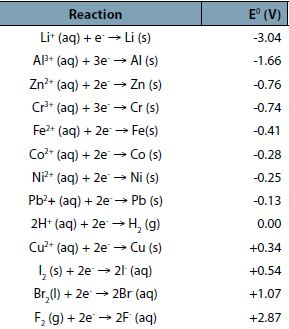# Standard Reduction Potentials

The electrode potential is an intensive property whose value is independent of the amount of species in the reaction. Thus, the electrode potential for the following half-reaction is the same for this half-reaction Cu2+ (aq) + 2e → Cu (s) is the same for this half-reaction 2 Cu2+ (aq) + 4e → 2Cu (s).

A voltage cannot be measured just for a half-cell but only for a complete cell. Therefore, to describe the reduction potential for a particular redox couple, the emf is measured for a cell where the other half-cell is a standard reference electrode. The standard reduction potential is in a category known as the standard cell potentials or standard electrode potentials. The standard cell potential is the potential difference between the cathode and anode.

A list of common standard reaction potentials is given below: